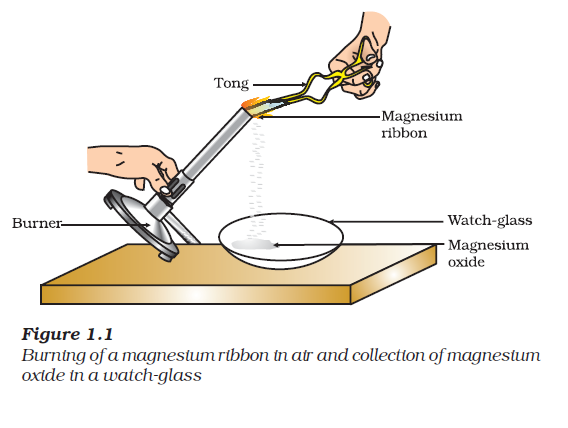Important points

 i) A complete chemical equation represents the reactants, products and their physical states symbolically.  ii) A chemical equation is balanced so that the numbers of atoms of each type involved in a chemical reaction are the same on the reactant and product sides of the equation. Equations must always be balanced.  iii) In a combination reaction two or more substances combine to form a new single substance.  iv) Decomposition reactions are opposite to combination reactions. In a decomposition reaction, a single substance decomposes to give two or more substances.  v) Reactions in which heat is given out along with the products are called exothermic reactions.  vi) Reactions in which energy is absorbed are known as endothermic reactions. vii) When an element displaces another element from its compound, a displacement reaction occurs. viii) Two different atoms or groups of atoms (ions) are exchanged in double displacement reactions. ix) Precipitation reactions produce insoluble salts.  x) Reactions also involve the gain or loss of oxygen or hydrogen by substances. + Oxidation is the gain of oxygen or loss of hydrogen.  + Reduction is the loss of oxygen or gain of hydrogen.

we can say that any of the following observations helps us to determine whether a chemical reaction has taken place –
1.  change in state
2.  change in colour
3.  evolution of a gas
4.  change in temperature.

Activity 1.1You must have observed that magnesium ribbon burns with a dazzling white flame and changes into a white powder.

This powder is magnesium oxide. It is formed due to the reaction between magnesium and oxygen present in the air.

Magnesium + Oxygen Magnesium oxide
(Reactants)                             (Product)

Balanced Chemical Equations

Law of conservation of massMass can neither be created nor destroyed in a chemical reaction. That is, the total mass of the elements present in the products of a chemical reaction has to be equal to the total mass of the elements present in the reactants.

In other words, the number of atoms of each element remains the same, before and after a chemical reaction. Hence, we need to balance a skeletal chemical equation.

balanced equation
3Fe + 4H2O Fe3O4 + 4H2

Writing Symbols of Physical States

3Fe(s) + 4H2O(g) Fe3O4(s) + 4H2(g)

To make a chemical equation more informative, the physical states of the reactants and products are mentioned along with their chemical formulae. The gaseous, liquid, aqueous and solid states of reactants and products are represented by the notations (g), (l), (aq) and (s), respectively. The word aqueous (aq) is written if the reactant or product is present as a solution in water.

TYPES OF CHEMICAL REACTIONS

1. Combination Reaction
2. Decomposition Reaction
3. Displacement Reaction
4. Double Displacement Reaction
5. Oxidation and Reduction

Combination Reaction

Such a reaction in which a single product is formed from two or more reactants is known as a combination reaction.

In simple language we can say that when two or more substances (elements or compounds) combine to form a single product, the reactions are called combination reactions.

Let us discuss some examples of combination reactions.

CaO (s)     +         H2O (l)      Ca(OH)2 (aq)

(Quick lime)                                  (Slaked lime)

(i) Burning of coal

C(s) + O2(g) CO2(g)

(ii) Formation of water from H2(g) and O2(g)

2H2(g) + O2(g) 2H2O(l)

Exothermic Chemical Reactions

Reactions in which heat is released along with the formation of products are called exothermic chemical reactions.

Examples of exothermic reactions are –

(i) Burning of natural gas

CH4(g) + 2O2 (g) → CO2 (g) + 2H2O (g)

(ii) Do you know that respiration is an exothermic process?

C6H12O6(aq) + 6O2(aq) → 6CO2(aq) + 6H2O(l) + energy

(Glucose)

The special name of this reaction is respiration.

(iii) The decomposition of vegetable matter into compost is also an example of an exothermic reaction.

Decomposition Reaction

Decomposition reactions are opposite to combination reactions. In a decomposition reaction, a single substance decomposes to give two or more substances.

CaCO3(s)   Heat    CaO(s) + CO2(g)

(Limestone)                     (Quick lime)

2FeSO4(s)  Heat →  Fe2O3(s) + SO2(g) + SO3(g)

(Ferrous sulphate)        (Ferric oxide)

When a decomposition reaction is carried out by heating, it is called thermal decomposition.

Decomposition reactions require energy either in the form of heat, light or electricity for breaking down the reactants.

Endothermic reactions

Reactions in which energy is absorbed are known as endothermic reactions.

Displacement Reaction

When an element displaces another element from its compound, displacement reaction occurs.

Fe(s) + CuSO4(aq) FeSO4(aq) + Cu(s)
(Copper sulphate)          (Iron sulphate)

In this reaction, iron has displaced or removed another element, copper, from copper sulphate solution. This reaction is known as displacement reaction.

Other examples of displacement reactions are

Zn(s) + CuSO4(aq) ZnSO4(aq) + Cu(s)
(Copper sulphate)         (Zinc sulphate)

Pb(s) + CuCl2(aq) PbCl2(aq) + Cu(s)

Zinc and lead are more reactive elements than copper. They displace copper from its compounds.

Double Displacement Reaction

Two different atoms or groups of atoms (ions) are exchanged in double displacement reactions.

Such reactions in which there is an exchange of ions between the reactants are called double displacement reactions.

Na2SO4(aq)          +    BaCl2(aq)             →       BaSO4(s)      +    2NaCl(aq)
(Sodium sulphate)     (Barium chloride)       (Barium  sulphate)      (Sodium chloride)

The white precipitate of BaSO4 is formed by the reaction of 2– SO4 and Ba2+. The other product formed is sodium chloride which remains in the solution.

Precipitate - Insoluble substance formed is known as a precipitate.

Precipitation ReactionAny reaction that produces a precipitate can be called a precipitation reaction.